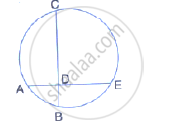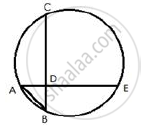Share

# In the Figure, Chords Ae and Bc Intersect Each Other at Point D. If ∠ Cde = 90°, Ab = 5 Cm, Bd = 4 Cm and Cd = 9 Cm Find De. - Mathematics

Course

#### Question

In the figure, chords AE and BC intersect each other at point D.
If ∠ CDE = 90°, AB = 5 cm, BD = 4 cm and CD = 9 cm
Find DE.#### SolutionJoin AB.

"AB" ^2 = "AD"^2  + "DB"^2

5^2 = "AD"^2 + 4^2

"AD"^2 =  25 - 16

"AD"^2 9

Chords AE and CB intersect each other at D inside the circle

AD x DE = BD x DC

3 × DE = 4 × 9

DE = 12 cm

Is there an error in this question or solution?